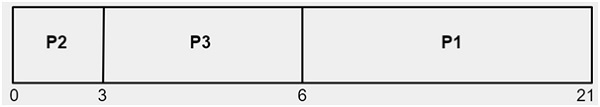# C Program for FCFS Scheduling

We are given with the n number of processes i.e. P1, P2, P3,.......,Pn and their corresponding burst times. The task is to find the average waiting time and average turnaround time using FCFS CPU Scheduling algorithm.

What is Waiting Time and Turnaround Time?

• Turnaround Time is the time interval between the submission of a process and its completion.

Turnaround Time = completion of a process – submission of a process

• Waiting Time is the difference between turnaround time and burst time

Waiting Time = turnaround time – burst time

What is FCFS Scheduling?

First Come, First Served (FCFS) also known as First In, First Out(FIFO) is the CPU scheduling algorithm in which the CPU is allocated to the processes in the order they are queued in the ready queue.

FCFS follows non-preemptive scheduling which mean once the CPU is allocated to a process it does not leave the CPU until the process will not get terminated or may get halted due to some I/O interrupt.

## Example

Let’s say, there are four processes arriving in the sequence as P2, P3, P1 with their corresponding execution time as shown in the table below. Also, taking their arrival time to be 0.

ProcessOrder of arrivalExecution time in msec
P1315
P213
P323

Gantt chart showing the waiting time of processes P1, P2 and P3 in the systemAs shown above,

The waiting time of process P2 is 0

The waiting time of process P3 is 3

The waiting time of process P1 is 6

Average time = (0 + 3 + 6) / 3 = 3 msec.

As we have taken arrival time to be 0 therefore turn around time and completion time will be same.

## Example

Input-:  processes = P1, P2, P3
Burst time = 5, 8, 12
Output-:
Processes  Burst    Waiting    Turn around
1          5        0           5
2          8        5           13
3          12       13          25
Average Waiting time = 6.000000
Average turn around time = 14.333333

## Algorithm

Start                                                                                                     Step 1-> In function int waitingtime(int proc[], int n, int burst_time[], int wait_time[])
Set wait_time = 0
Loop For i = 1 and i < n and i++
Set wait_time[i] = burst_time[i-1] + wait_time[i-1]
End For
Step 2-> In function int turnaroundtime( int proc[], int n, int burst_time[], int wait_time[], int tat[])
Loop For  i = 0 and i < n and i++
Set tat[i] = burst_time[i] + wait_time[i]
End For
Step 3-> In function int avgtime( int proc[], int n, int burst_time[])
Declare and initialize wait_time[n], tat[n], total_wt = 0, total_tat = 0;
Call waitingtime(proc, n, burst_time, wait_time)
Call turnaroundtime(proc, n, burst_time, wait_time, tat)
Loop For  i=0 and i<n and i++
Set total_wt = total_wt + wait_time[i]
Set total_tat = total_tat + tat[i]
Print process number, burstime wait time and turnaround time
End For
Print "Average waiting time =i.e. total_wt / n
Print "Average turn around time = i.e. total_tat / n
Step 4-> In int main()
Declare the input int proc[] = { 1, 2, 3}
Declare and initialize n = sizeof proc / sizeof proc
Declare and initialize burst_time[] = {10, 5, 8}
Call avgtime(proc, n, burst_time)
Stop

## Example

Live Demo

#include <stdio.h>
// Function to find the waiting time for all processes
int waitingtime(int proc[], int n,
int burst_time[], int wait_time[]) {
// waiting time for first process is 0
wait_time = 0;
// calculating waiting time
for (int i = 1; i < n ; i++ )
wait_time[i] = burst_time[i-1] + wait_time[i-1] ;
return 0;
}
// Function to calculate turn around time
int turnaroundtime( int proc[], int n,
int burst_time[], int wait_time[], int tat[]) {
// calculating turnaround time by adding
// burst_time[i] + wait_time[i]
int i;
for ( i = 0; i < n ; i++)
tat[i] = burst_time[i] + wait_time[i];
return 0;
}
//Function to calculate average time
int avgtime( int proc[], int n, int burst_time[]) {
int wait_time[n], tat[n], total_wt = 0, total_tat = 0;
int i;
//Function to find waiting time of all processes
waitingtime(proc, n, burst_time, wait_time);
//Function to find turn around time for all processes
turnaroundtime(proc, n, burst_time, wait_time, tat);
//Display processes along with all details
printf("Processes  Burst   Waiting Turn around ");
// Calculate total waiting time and total turn
// around time
for ( i=0; i<n; i++) {
total_wt = total_wt + wait_time[i];
total_tat = total_tat + tat[i];
printf(" %d\t  %d\t\t %d \t%d", i+1, burst_time[i], wait_time[i], tat[i]);
}
printf("Average waiting time = %f", (float)total_wt / (float)n);
printf("Average turn around time = %f", (float)total_tat / (float)n);
return 0;
}
// main function
int main() {
//process id's
int proc[] = { 1, 2, 3};
int n = sizeof proc / sizeof proc;
//Burst time of all processes
int burst_time[] = {5, 8, 12};
avgtime(proc, n, burst_time);
return 0;
}

## Output

Processes  Burst    Waiting    Turn around
1          5        0           5
2          8        5           13
3          12       13          25
Average Waiting time = 6.000000
Average turn around time = 14.333333